Vol 17, No 7 (2017) / Yadav

Preferred alignments of angular momentum vectors of galaxies in six dynamically unstable Abell clusters

Preferred alignments of angular momentum vectors of galaxies in six dynamically unstable Abell clusters

Yadav Shiv N.1, , Aryal Binil1, , Saurer Walter2

Central Department of Physics, Tribhuvan University, Kirtipur, Nepal
Institute of Astro-particle Physics, Innsbruck University, A-6020 Innsbruck, Austria

† Corresponding author. E-mail: ysibnarayan@yahoo.com

Abstract: Abstract

A spatial orientation of angular momentum vectors of galaxies in six dynamically unstable Abell clusters (S1171, S0001, A1035, A1373, A1474 and A4053) is studied. For this, two-dimensional observed parameters (e.g., positions, diameters and position angles) are converted into three-dimensional (3D) rotation axes of the galaxy using the ‘position angle - inclination’ method. The expected isotropic distribution curves for angular momentum vectors are obtained by performing random simulations. The observed and expected distributions are compared using several statistical tests. No preferred alignments of angular momentum vectors of galaxies are noticed in all six dynamically unstable clusters, supporting the hierarchy model of galaxy formation. These clusters have a larger value of velocity dispersion. However, local effects are noticed in the clusters that have substructures in the 1D-3D number density maps.

Keywords: galaxies: evolution;galaxies: clusters: general;astronomical databases: miscellaneous

1 Introduction

The formation of a galaxy cluster is one of the major unsolved problems of modern astrophysics. The process by which larger structures (e.g., clusters, superclusters) are formed through the continuous merging of smaller structures (e.g., galaxies and galaxy groups) is called hierarchical clustering, which is supported by the concordance model (ΛCDM). The study of the preferred alignment of angular momentum vectors of galaxies in clusters is one of the most effective ways of testing the concordance model. Godłowski et al. () described the Li () model and showed the relation between angular momenta and masses of the large scale structures. This relationship was observationally tested by several authors (Godłowski et al. ; Hu et al. ; Aryal & Saurer ; Aryal et al. , ; Godłowski et al. , Godłowski ) and they found vanishing angular momenta for less massive structures but non-vanishing cases for larger structures.

Aryal et al. () studied preferred alignments of angular momentum vectors of galaxies in six rotating clusters (A954, A1139, A1399, A2162, A2169 and A2366) that are dynamically stable and have a single peak in one-dimensional (1D)-three-dimensional (3D) number density maps. These clusters have no substructures. They found a random orientation of angular momentum vectors of galaxies in all six clusters, supporting the hierarchy model (Peebles ).

In the present work we intend to study the preferred alignments of angular momentum vectors of galaxies in six Abell clusters, namely S1171, S0001, A1035, A1373, A1474 and A4053, that have multiple peaks in 1D-3D number density maps with a larger value of velocity dispersion. These clusters have substructures. We intend to answer the following: (1) Does the orientation of angular momentum vectors of galaxies that have substructures favor hierarchical clustering? (2) Do the clusters with large velocity dispersion prefer a random orientation of angular momentum vectors of galaxies? And finally, (3) does substructure formation cause large velocity dispersion in the cluster? Our aim is to compare results with the concordance model. The database and methods are described in Sections 2 and 3 respectively. Our results and conclusions are presented in Sections 4 and 5 respectively.

2 Database

Hwang & Lee (, HL hereafter) identified six rotating clusters (A954, A1139, A1399, A2162, A2169 and A2366) that are in dynamical equilibrium and show a single peak in 1D-3D number density maps. In addition, six dynamically unstable clusters (S1171, S0001, A1035, A1373, A1474 and A4053) that have multiple number-density peaks in 1D-3D maps with a large velocity dispersion are present. After investigating substructure using the Dressler-Schectman method, HL classified rotating clusters into two categories: (1) clusters with a single number density peak which hence are in dynamical equilibrium and (2) clusters with multiple number-density peaks which are dynamically unstable. In both cases, clusters have a very large value of velocity dispersion. In this paper we study the preferred alignments of angular momentum vectors of galaxies in Abell clusters S1171, S0001, A1035, A1373, A1474 and A4053.

Table 1 lists the database (positions, BM type classification, mean redshift, velocity dispersion, number of galaxies in the cluster and its morphology) of six clusters (HL; Hwang private communication in 2011) used for this study.

Abell R.A. Dec. BM-type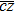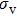N Morphology
(J2000) (J2000)   (km s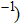(km s(1) (2) (3) (4) (5) (6) (7) (8)
S1171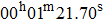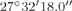II 8377 646 42 Spherical
S0001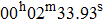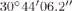I 8815 577 51 Elongated
A1035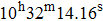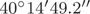II–III 21753 1825 97 Spherical
A1373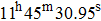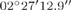III 37595 1768 48 Elongated
A1474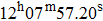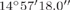III 24151 714 60 Elongated
A4053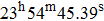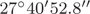III 20691 1366 76 Elongated

Table 1Database of six clusters that have multiple number-density peaks in 1D–3D maps (HL). The first column lists the Abell name followed by its position (Abell et al. ). The fourth-eighth columns give BM type cluster morphology (Bautz & Morgan ), mean radial velocity (), velocity dispersion (), and number (N) of galaxies in the cluster and its morphology, as given in HL..

3 Method

The position angle (PA)-inclination method is used to convert given two-dimensional (2D) parameters (positions, diameters, PAs) into 3D ones (galaxy rotation axes: angular momentum vectors and their projections) (Flin & Godlowski ). The expected isotropic distribution curves for angular momentum vectors and their projections are determined by performing a random simulation (Aryal & Saurer ). The observed distributions are compared with the expected ones using various statistical tests.

3.1 Observed Distribution: Angular Momentum Vectors of Galaxies

The angular momentum vectors (θ) of galaxies and their projections (ϕ) to the galactic (G) and supergalactic (S) planes are obtained by using the method described by Flin & Godlowski (). For this, the SDSS/2dFGRS databases (positions, PAs and diameters) provided by Hwang (private communication in 2011) are used. In previous works (Godlowski ; Baier et al. ; Hu et al. and the references therein), authors have studied the preferred alignments of galaxies in clusters with respect to the galactic or supergalactic (or both) system. The formulae to obtain angular momentum vectors (θ) and their projection (ϕ) in the S-system are as follows (Flin & Godlowski ):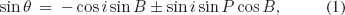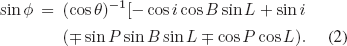The inclination angle (i) is the angle between the line-of-sight and normal to the plane of the galaxy. This angle can be calculated using the Holmberg () formula where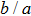is the measured axial ratio and q is the intrinsic flatness of disk galaxies. Since the morphological information on galaxies is not known, the value of intrinsic flatness (q) is assumed to be 0.2 (Aryal et al. ; Godłowski ). The parameters L, B and P are the supergalactic longitude and latitude, and PA, respectively.

Equations (1) and (2) give two solutions for a galaxy. The reason for this is the approaching and receding sides of a galaxy, which cannot be identified in our database. Therefore, there are four solutions for the preferred alignments of a galaxy: two each for angular momentum vectors and their projections. We count all these possibilities independently in the analysis.

3.2 Expected Distribution: Numerical Simulation

Aryal & Saurer () performed random simulations imposing various types of selections in the database and concluded that any selections can cause changes in the expected isotropic distribution curves for both angular momentum vectors (polar angles) and their projections (azimuthal angles). We noticed the following selection effects in our database: (1) the positions of galaxies in the clusters are inhomogeneous; (2) the PAs of face-on cases (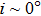) are mostly unknown and (3) a deficiency in high inclination (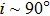) galaxies. We perform the random simulation method proposed by Aryal & Saurer () in order to find expected isotropic distribution curves (θ and ϕ) by removing the above mentioned selection effects. We apply the cosmological principle by assuming an isotropic distribution of angular momentum vectors for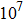virtual galaxies and use Equations (1) and (2) in the random simulation. Therefore, the inclination angle (i) and latitude (B) are distributed as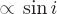and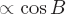, respectively and the variables longitude (L) and PA are distributed randomly (Aryal & Saurer ).

3.3 Statistical Tests

We apply chi-square, autocorrelation, Fourier (Godlowski ), Kolmogorov-Smirnov (K-S) (Press et al. ) and Kuiper-V (Kuiper ) tests to discriminate anisotropy from isotropy in the observed and expected distributions. Details about these statistical tests are given in the appendix of Aryal et al. (). The limits for anisotropy are as follows:

chi-square probability (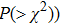< 0.050,

autocorrelation coefficient (C/C(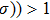,

first order Fourier coefficient (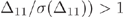,

Fourier probability (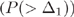< 0.150,

K-S=1,

Kuiper-V = 1.

In the last two statistical tests, the null hypothesis (isotropy) is represented by “0” which is not rejected at the chosen significance level, whereas the value “1” designates that the null hypothesis is rejected (anisotropy).

The first order Fourier coefficient (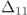) provides information regarding preferred alignments. A positive (negative) value of first order Fourier coefficient () in the θ-distribution suggests that the angular momentum vectors of galaxies tend to orient parallel (perpendicular) with respect to the reference coordinate system. Similarly, a positive (negative)in the ϕ-distribution indicates that the projections of angular momentum vectors of galaxies in the galactic (G) or supergalactic (S) planes tend to point radially (tangentially) towards the center of the reference coordinate system.

4 Results

Table 2 shows the values of statistical parameters with respect to galactic (G) and supergalactic (S) coordinate systems. In this section we discuss polar (θ) and azimuthal (ϕ) angle distributions of galaxies in each cluster separately.

Abell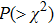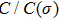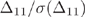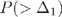K-S Kuiper-V
G/S G/S G/S G/S G/S G/S
(1) (2) (3) (4) (5) (6) (7)
Polar Angle
S1171 0.959/0.820 –0.5/+0.1 +0.0/–0.6 0.999/0.805 0/0 0/0
S0001 0.554/0.779 –0.4/+0.7 –0.6/+1.2 0.798/0.435 0/0 0/0
1035 0.721/0.795 +0.1/–1.0 +0.4/–0.5 0.864/0.868 0/0 0/0
1373 0.495/0.553 +0.7/–0.9 –0.2/–0.2 0.796/0.964 0/0 0/0
1474 0.012/0.610 –1.8/+0.2 +1.1/–0.9 0.380/0.501 0/0 0/0
4053 0.718/0.937 –1.3/+0.1 –0.4/–0.6 0.900/0.825 0/0 0/0
Azimuthal Angle
S1171 0.264/0.759 +0.1/–0.2 +2.0/–0.4 0.141/0.903 0/0 0/0
S0001 0.267/0.914 –0.8/–0.1 –1.8/+0.3 0.185/0.928 0/0 0/0
1035 0.851/0.154 +0.4/–1.7 –1.5/–0.2 0.296/0.883 0/0 0/0
1373 0.041/0.964 +0.7/0.1 –2.0/+0.1 0.019/0.980 0/0 0/0
1474 0.000/0.271 –0.7/–0.2 +0.9/+0.6 0.118/0.646 0/0 0/0
4053 0.064/0.104 –0.6/–0.7 –2.2/–1.2 0.095/0.333 0/0 0/0

Table 2Statistical values for both θ and ϕ distributions. The Abell number of the cluster, values of chi-square probability () and autocorrelation coefficients (C/C(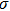)) are given in the first three columns. The fourth-seventh columns list the values of first order Fourier coefficient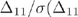), first order Fourier probability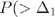), and the results of K-S and Kuiper-V tests, respectively..

4.1 Abell S1171

Abell S1171 is the spherically shaped nearby (= 8377 km s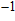) cluster in our database (Table 1). HL noticed that a few galaxies have large velocity deviations (cz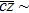1400 km s) from the cluster’s main body (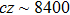km s). The position and radial velocity (cz) distributions of member galaxies show a bimodal velocity distribution (Fig. 1(a)). A large number of substructures with central condensation can be seen in the number density map (Fig. 2(b)).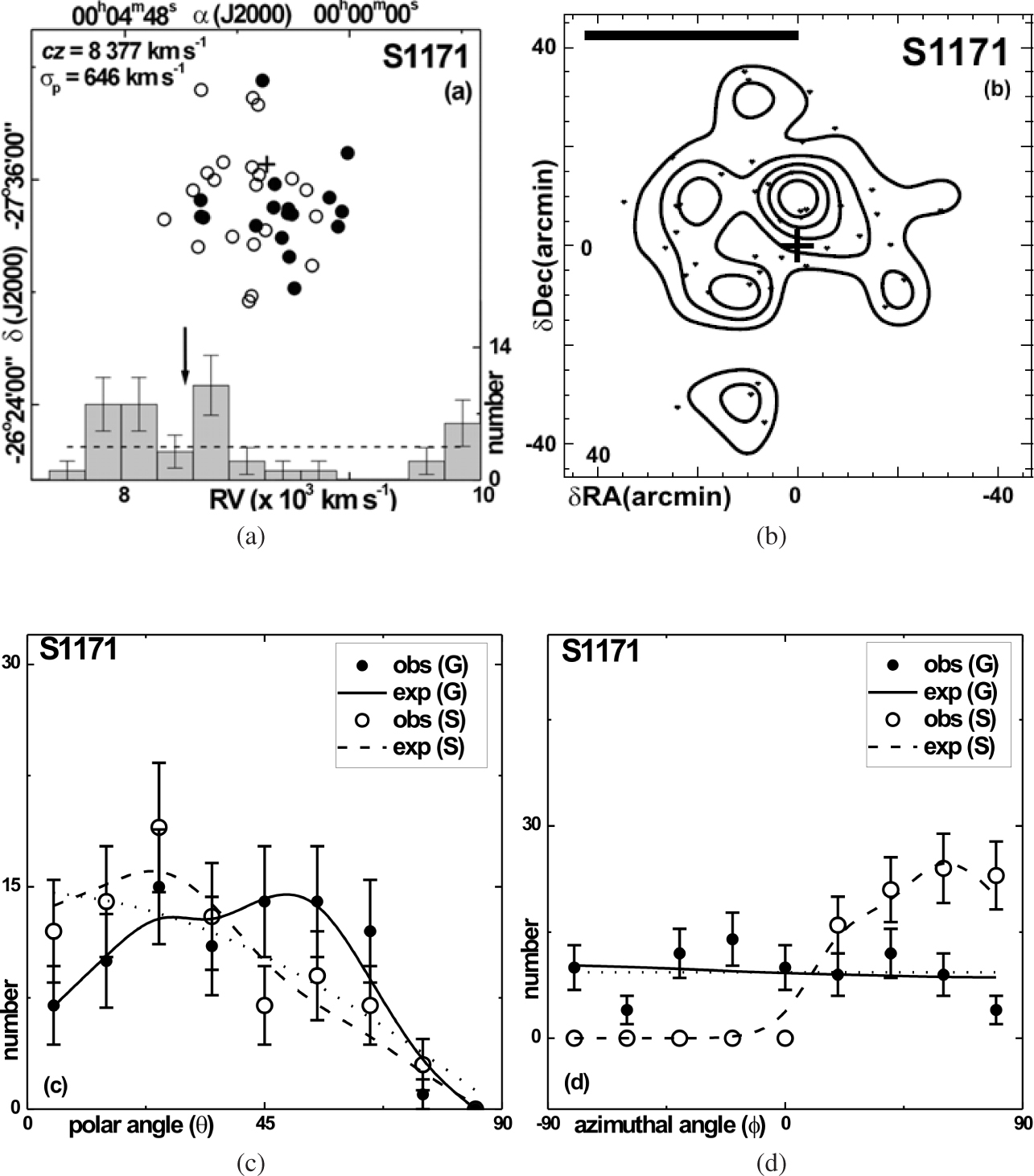Fig. 1 (a) Distribution of galaxies in Abell S1171. The galaxies having radial velocity (cz) < (>)of the cluster are represented by solid (hollow) circles. The dashed line and an arrow represent the average distribution of cz of galaxies and the clusterrespectively. (b) Number density map: the member galaxies are represented by dots and the number density contours are overlaid. The cross and thick horizontal bar indicate the cluster center and the physical size of 1 Mpc respectively. (c) The polar (θ) and (d) azimuthal (ϕ) angle distributions of galaxies with respect to the G- (solid line) and S- (dashed line) coordinate systems. The cosine and average distributions (dotted line) are shown for the comparison. The statistical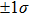error bars are shown for the observed counts.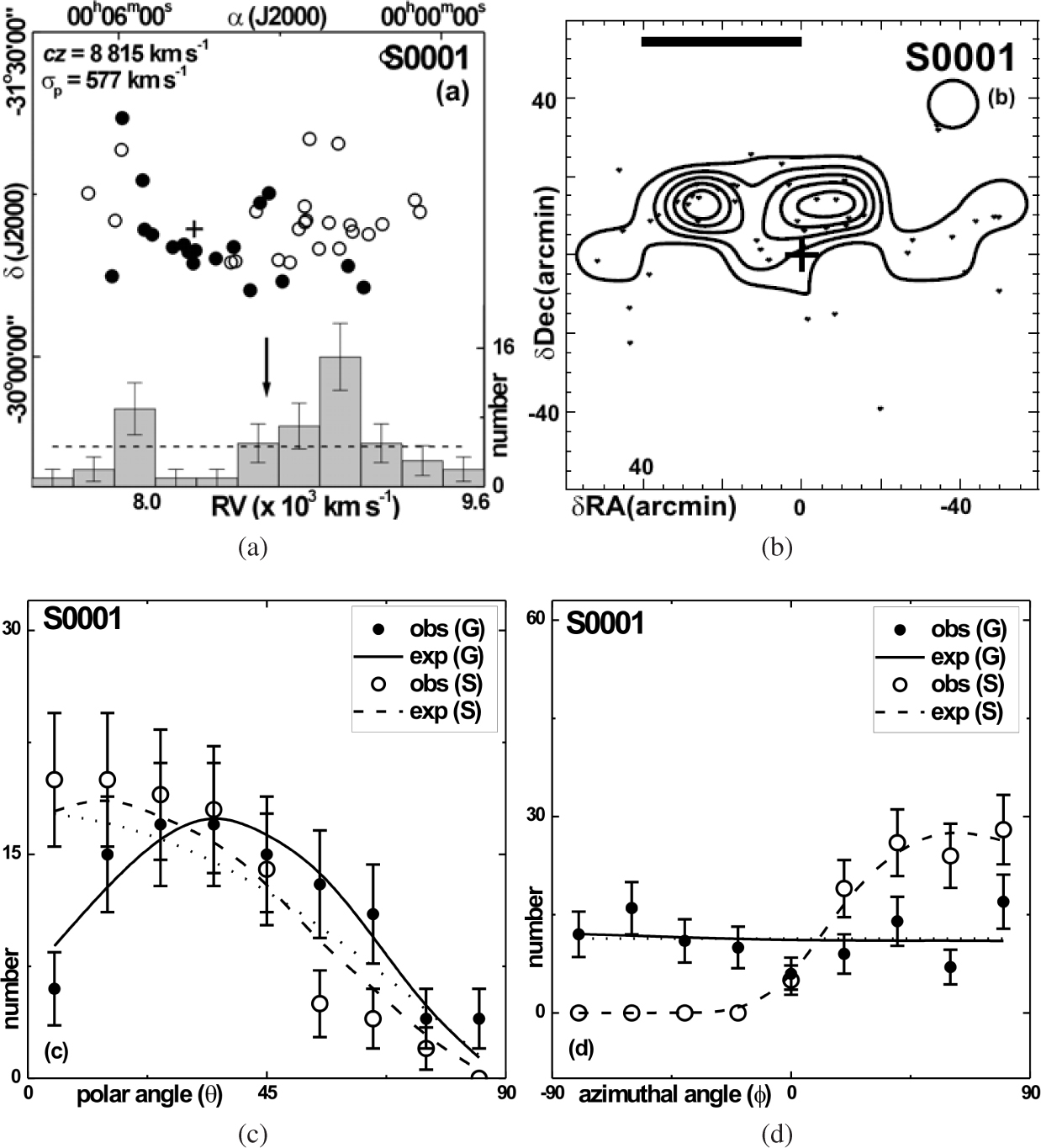Fig. 2 The cluster Abell S0001: (a) the scatter plot, (b) number density map, (c) θ and (d) ϕ distributions of galaxies. Symbols and explanations are the same as in Fig. 1.

Figure 1(c) and (d) shows the polar (θ) and azimuthal angle (ϕ) distributions of member galaxies in S1171. The solid and dashed curves represent the expected isotropic distribution curves obtained from random simulation. The polar angle,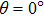(90°) corresponds to the angular momentum vector that tends to lie parallel (perpendicular) to the galactic/supergalactic plane. All six statistical parameters suggest isotropy in the θ-distribution (see Table 2). No preferred alignment of angular momentum vectors of galaxies is observed with respect to the G- and S-coordinate systems. Therefore, a random orientation of angular momentum vectors of galaxies is noticed in the cluster.

In the ϕ-distribution, 0° corresponds to the projections of angular momentum vectors that tend to point radially towards the center of the reference coordinate system (center of the Milky Way in G-system and Virgo Cluster center in S-system). No humps or dips are observed in Figure 1(d), suggesting no preferred alignments. It can be concluded that the galaxies with a large velocity dispersion cause the cluster to be dynamically unstable as discussed by Godłowski ().

4.2 Abell S0001

HL noticed substructures in 2D and 3D number density maps. A low-velocity tail at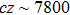km swhich is far from the mean radial velocity of the cluster can be seen in Figure 2(a). Figure 2(b) shows two widely separated local minima, which strongly suggest that the cluster might not be in dynamical equilibrium.

All six statistical parameters show isotropy in both the θ- and ϕ-distributions (Table 2). A very good correlation between the expected and observed distribution can be seen in the histograms (Fig. 2(c),(d)), suggesting a random orientation of angular momentum vectors of galaxies. No preferred alignments of angular momentum vectors of galaxies in the cluster Abell S0001 are found.

4.3 Abell 1035

This cluster has the largest velocity dispersion with two subclusterings along north and south (Fig. 3(a),(b)). HL found substructures in all maps (1D, 2D and 3D), suggesting a large but unequal velocity dispersion causing it to be dynamically unstable. Using a photometric database (Lauberts ), Aryal & Saurer () studied the preferred alignments in this cluster and found that the angular momentum vectors of galaxies tend to lie in the Local Supercluster (LSC) plane, supporting the pancake model (Doroshkevich ) of galaxy formation. HL found that the angle between rotation axes and the LSC plane is about 56°, suggesting a bimodal distribution.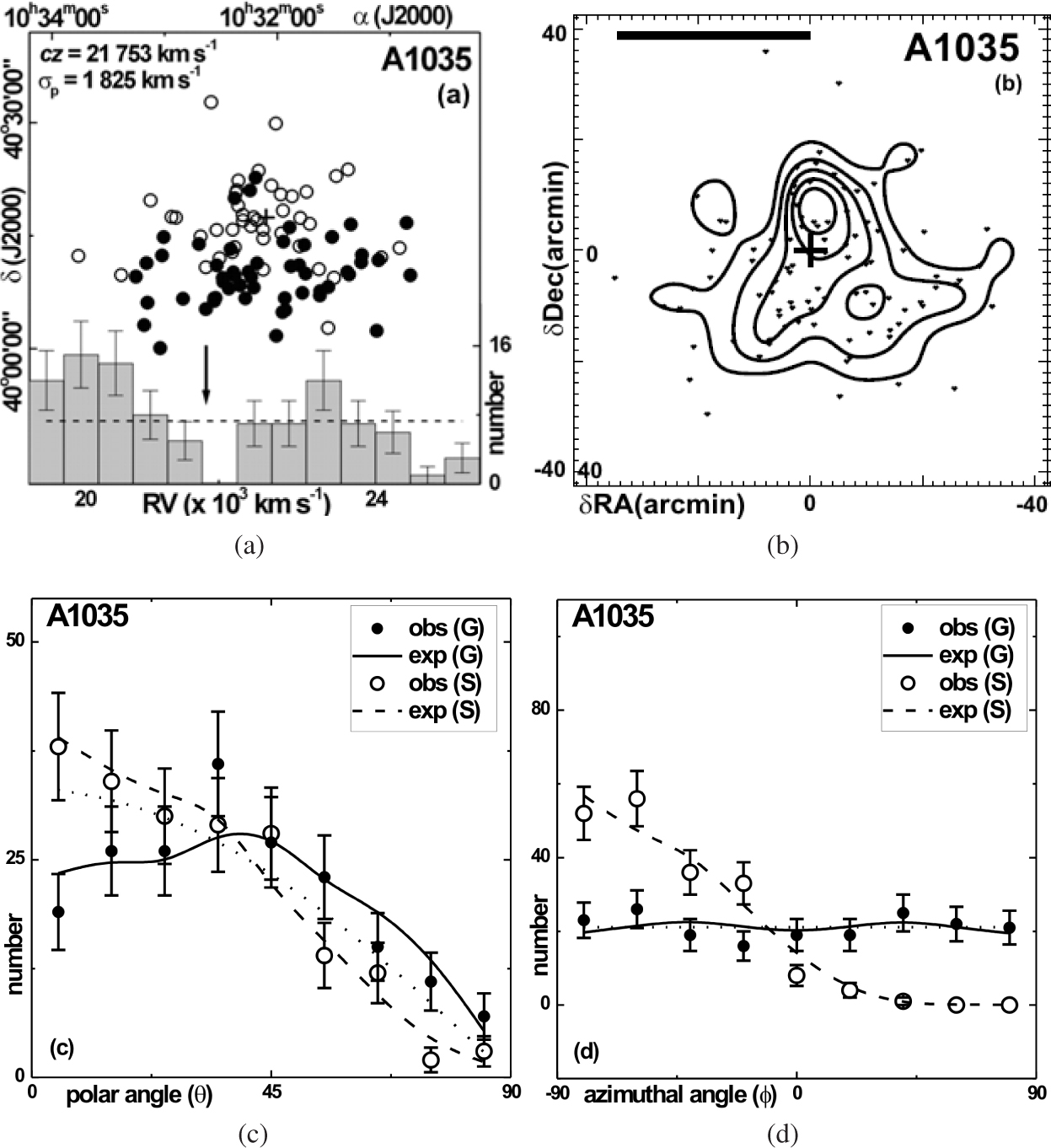Fig. 3 The cluster Abell A1035: (a) the scatter plot, (b) number density map, (c) θ and (d) ϕ distributions of galaxies. Symbols and explanations are the same as in Fig. 1.

In the present study we used spectroscopic databases (SDSS and 2dFGRS) and verified the prediction made by HL by observing a significant hump at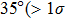) in the θ-distribution (Fig. 3(c)), supporting a bimodal distribution: angular momentum vectors of galaxies tend to lie both parallel and perpendicular with respect to the plane of the Milky Way. In the ϕ-distribution, isotropy is noticed (Fig. 3(d)), suggesting that the choice of coordinate system is independent of preferred alignments.

4.4 Abell 1373

This cluster is the most distant (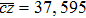km s) with bimodal velocity distribution spatially separated by the rotation axes (Fig. 4(a)). The number density map is similar to that of the cluster Abell 1035, i.e., substructure is seen in 1D-3D (HL).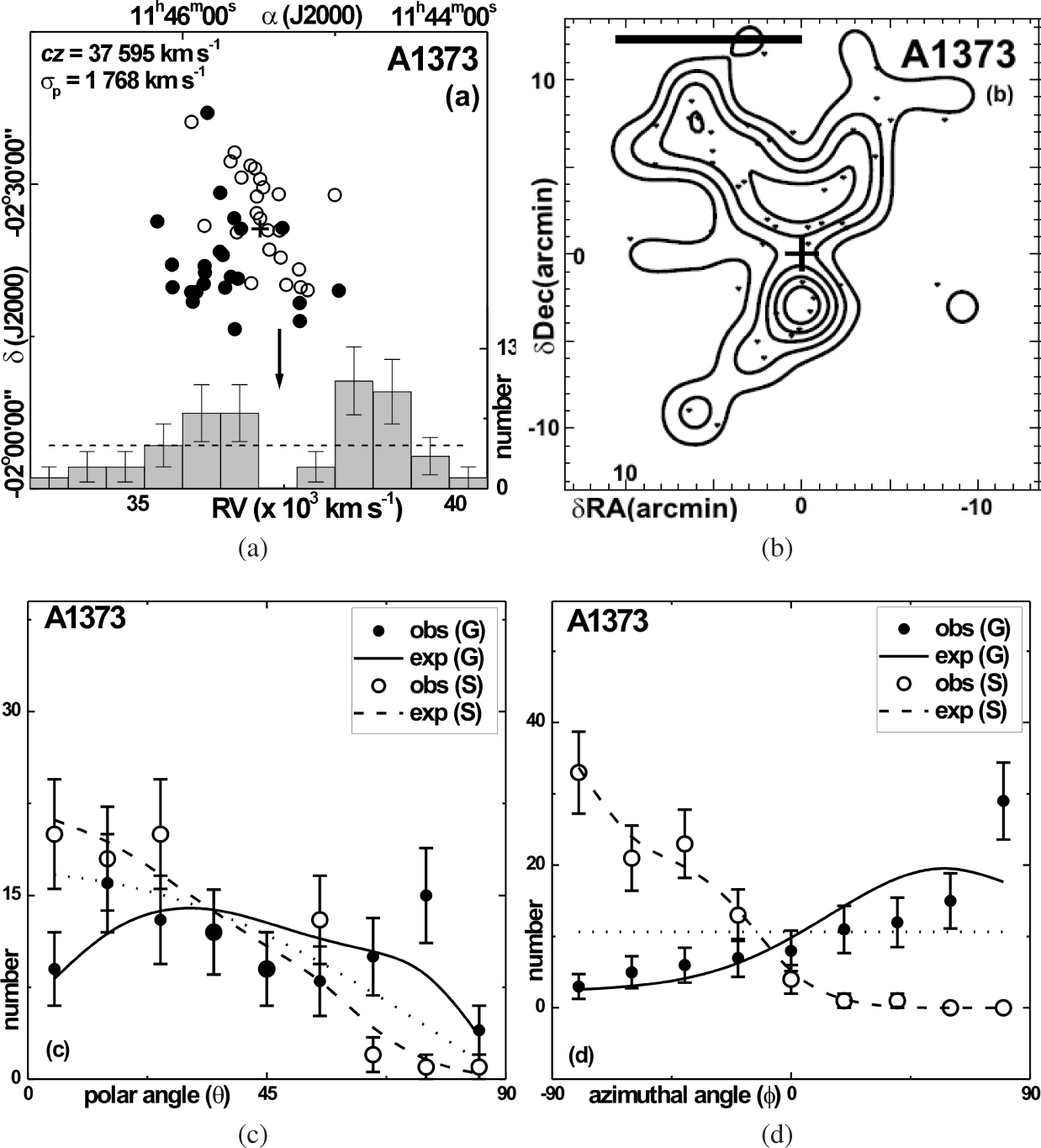Fig. 4 The cluster Abell A1373: (a) the scatter plot, (b) number density map, (c) θ and (d) ϕ distributions of galaxies. Symbols and explanations are the same as in Fig. 1.

All statistical tests suggest isotropy in both the θ- and ϕ-distributions, advocating the hierarchy model (Peebles ) of galaxy evolution. In the θ-distribution, a significant hump at 75° (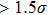) can be seen (Fig. 4(c)). In addition, a dip at 45° (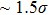) is followed by the hump. Therefore, a local tidal effect, probably due to substructure formation, is noticed. In the ϕ-distribution, a hump at 85° (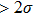) and dips at 65° () and 75° () support the local effect (Fig. 4(d)).

4.5 Abell 1474

Einasto et al. () studied the Virgo-Coma Supercluster and concluded that Abell 1474 is a member cluster of that supercluster. HL noticed three substructures in the number density map (Fig. 5(b)), suggesting a dynamically unstable rotating cluster which is not in dynamical equilibrium because of a large value of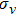.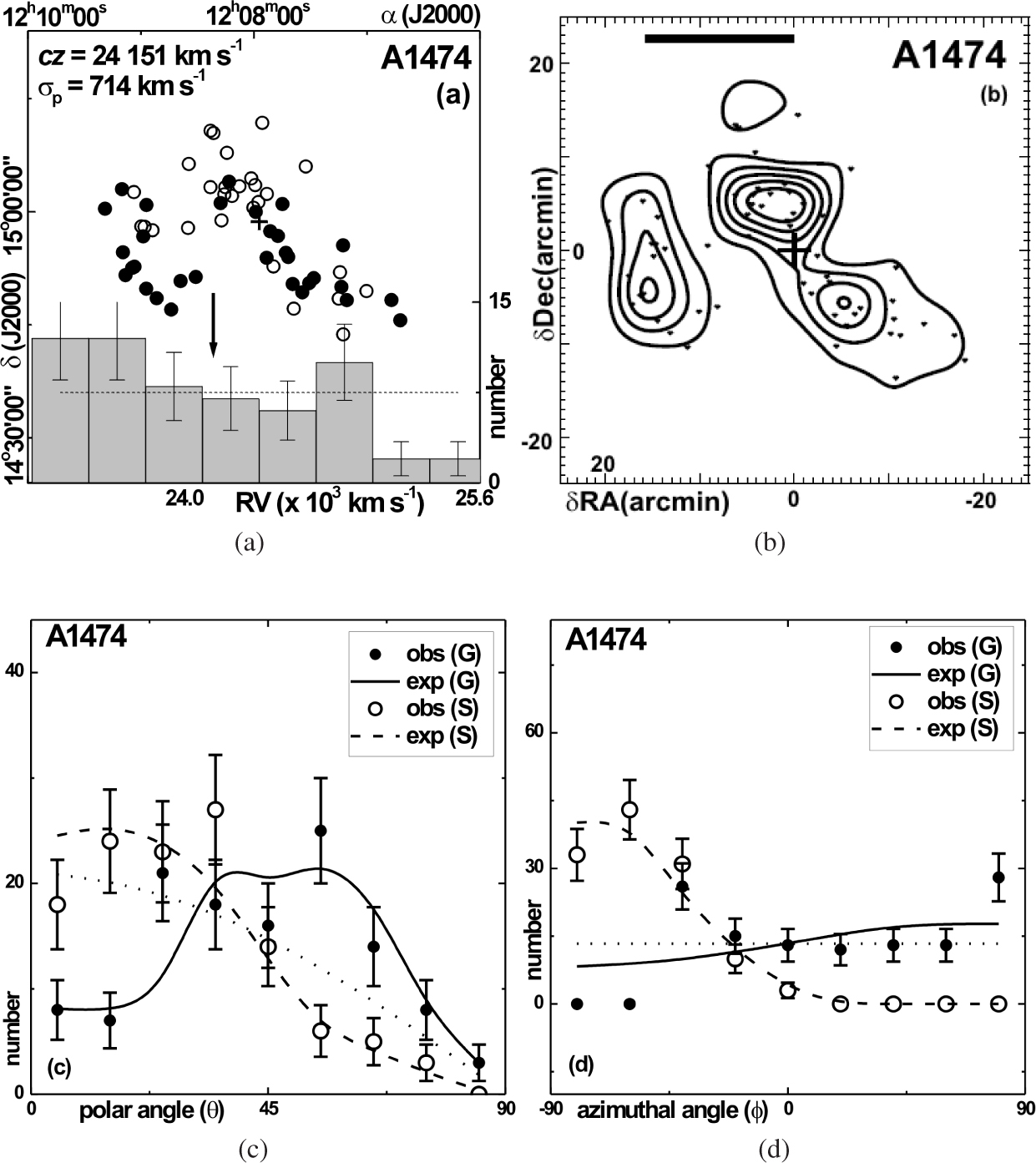Fig. 5 The cluster Abell A1474: (a) the scatter plot, (b) number density map, (c) θ and (d) ϕ distributions of galaxies. Symbols and explanations are the same as in Fig. 1.

The chi-squared and autocorrelation tests show anisotropy in the θ-distribution, whereas isotropy is noticed in the Fourier, K-S and Kuiper-V tests. A hump at 25° (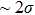) and a dip at 45° (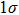) suggest a local tidal effect because of the subclustering (Fig. 5(c)). In the ϕ-distribution, significant humps at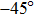(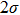) and 90° (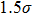) support it (Fig. 5(d)). Therefore, the spatial orientation of galaxies in the cluster Abell 1474 shows a weak preference in the alignments.

4.6 Abell 4053

Porter & Raychaudhury () reported that the cluster A4053 is a member of the Pisces-Cetus Supercluster. HL found that the cluster shows substructures in 1D and 3D maps. The number density map (Fig. 6(b)) shows elongation along the north-east and south-west directions.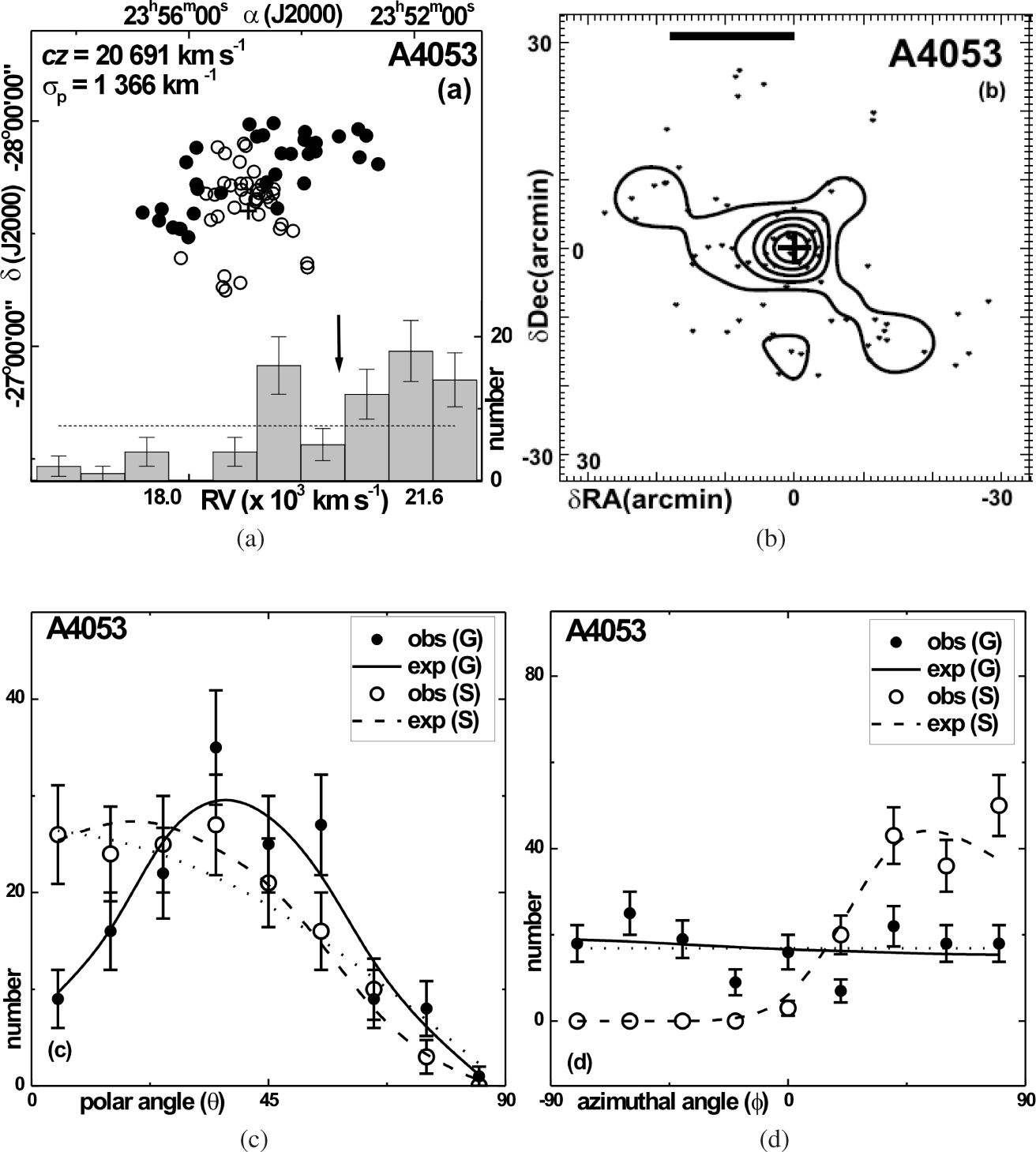Fig. 6 The cluster Abell A4053: (a) the scatter plot, (b) number density map, (c) θ and (d) ϕ distributions of galaxies. Symbols and explanations are the same as in Fig. 1.

In the histogram of polar (θ) angle distribution, a good agreement between the expected and observed distribution is noticed, suggesting no preferred alignments (Fig. 6(c)). All statistical tests support this. In the ϕ-distribution, humps (−70°, 50°) and dips (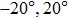) at thelevel are significant (Fig. 6(d)), suggesting anisotropy. Statistical tests support this result. Therefore, projections of angular momentum vectors of galaxies in A4053 tend to be oriented perpendicularly towards the plane of the Milky Way, whereas no preference is noticed with respect to the Virgo Cluster center. Therefore, a local effect cannot be ruled out in a cluster which has multiple number-density peaks and a large value of velocity dispersion.

5 Conclusions

The preferred alignments of angular momentum vectors of galaxies in six clusters having multiple number-density peaks with a spatial segregation of high- and low-velocity galaxies are studied. We adopted the ‘position angle - inclination’ method (Flin & Godlowski ) to compute 3D parameters (polar and azimuthal angles of galaxy rotation axes) using 2D observed parameters (e.g., positions, diameters, PA). To remove selection effects from the database, a numerical simulation is performed, as proposed by Aryal & Saurer (). The observed and expected isotropic distributions are compared using five statistical tests, namely chi-square, autocorrelation, Fourier, K-S and Kuiper-V.

In general, no preferred alignment is noticed for all six clusters, supporting the hierarchy model as predicted by Peebles (). However, local effects are noticed in the clusters that have substructures in 1D, 2D and 3D analysis (HL). Therefore, a large value of velocity dispersion with substructures in the clusters does not lead their galaxies to support the pancake (Doroshkevich ) or primordial vorticity theory (Ozernoi ). A very good correlation between the hierarchy (Peebles ) and Li model (Li ) is found, as in our previous work (Aryal et al. ). Therefore, vanishing angularmomenta favor the formation of substructures in clusters that have large velocity dispersion. The preferred alignment is found to increase with richness of the cluster. Therefore, it can be interpreted as an effect from the mechanism of tidal forces (Heavens & Peacock ; Catelan & Theuns ; Stephanovich & Godłowski ), but also is in agreement with the Li () model in which galaxies form in the rotating universe.

Tidal torque naturally arises in the hierarchical clustering scenario and hence the distribution of angular momentum vectors of galaxies becomes random. However, a tidal torque shear tensor (due to the effect of gravity) can cause a local preference in angular momentum vectors as predicted by Lee () and Trujillo et al. ().

 Abell G. O. Corwin H. G. Jr. Olowin R. P. 1989 ApJS 70 1 Aryal B. Bhattarai H. Dhakal S. Rajbahak C. Saurer W. 2013 MNRAS 434 1939 Aryal B. Kafle P. R. Saurer W. 2008 MNRAS 389 741 Aryal B. Paudel S. Saurer W. 2007 MNRAS 379 1011 Aryal B. Saurer W. 2000 AȦA 364 L97 Aryal B. Saurer W. 2006 MNRAS 366 438 Baier F. W. Godłowski W. MacGillivray H. T. 2003 A&A 403 847 Bautz L. P. Morgan W. W. 1970 ApJ 162 L149 Catelan P. Theuns T. 1996 MNRAS 282 436 Doroshkevich A. G. 1973 Astrophys. Lett. 14 11 Einasto M. Einasto J. Tago E. Müller V. Andernach H. 2001 AJ 122 2222 Flin P. Godlowski W. 1986 MNRAS 222 525 Godlowski W. 1993 MNRAS 265 874 Godłowski W. Szydłowski M. Flin P. Biernacka M. 2003 General Relativity and Gravitation 35 907 Godłowski W. Szydłowski M. Flin P. 2005 General Relativity and Gravitation 37 615 Godłowski W. Piwowarska P. Panko E. Flin P. 2010 ApJ 723 985 Godłowski W. 2011a Acta Physica Polonica B 42 2323 Godłowski W. 2011b International Journal of Modern Physics D 20 1643 Godłowski W. 2012 ApJ 747 7 Heavens A. Peacock J. 1988 MNRAS 232 339 Holmberg E. 1946 Meddelanden fran Lunds Astronomiska Observatorium Serie II 117 3 Hu F. X. Wu G. X. Song G. X. Yuan Q. R. Okamura S. 2006 Ap&SS 302 43 Hwang H. S. Lee M. G. 2007 ApJ 662 236 Kuiper N. H. 1960 Indagationes Mathematicae (Proceedings) 63 38 Lauberts A. 1982 ESO/Uppsala Survey of the ESO(B) Atlas Garching European Southern Observatory (ESO) 1982 Lee J. 2004 ApJ 614 L1 Li L.-X. 1998 General Relativity and Gravitation 30 497 Ozernoi L. M. 1978 IAU Symposium 79 Large Scale Structures in the Universe Longair M. S. Einasto J. 427 Peebles P. J. E. 1969 ApJ 155 393 Porter S. C. Raychaudhury S. 2005 MNRAS 364 1387 Press W. H. Teukolsky S. A. Vetterling W. T. Flannery B. P. 1992 Numerical Recipes in CN The Art of Scientific Computing 2 Cambridge Cambridge Univ. Press Stephanovich V. Godłowski W. 2015 ApJ 810 167 Trujillo I. Carretero C. Patiri S. G. 2006 ApJ 640 L111
Cite this article: Yadav Shiv N., Aryal Binil, Saurer Walter. Preferred alignments of angular momentum vectors of galaxies in six dynamically unstable Abell clusters. Res. Astron. Astrophys. 2017; 7:064.

Refbacks

• There are currently no refbacks.